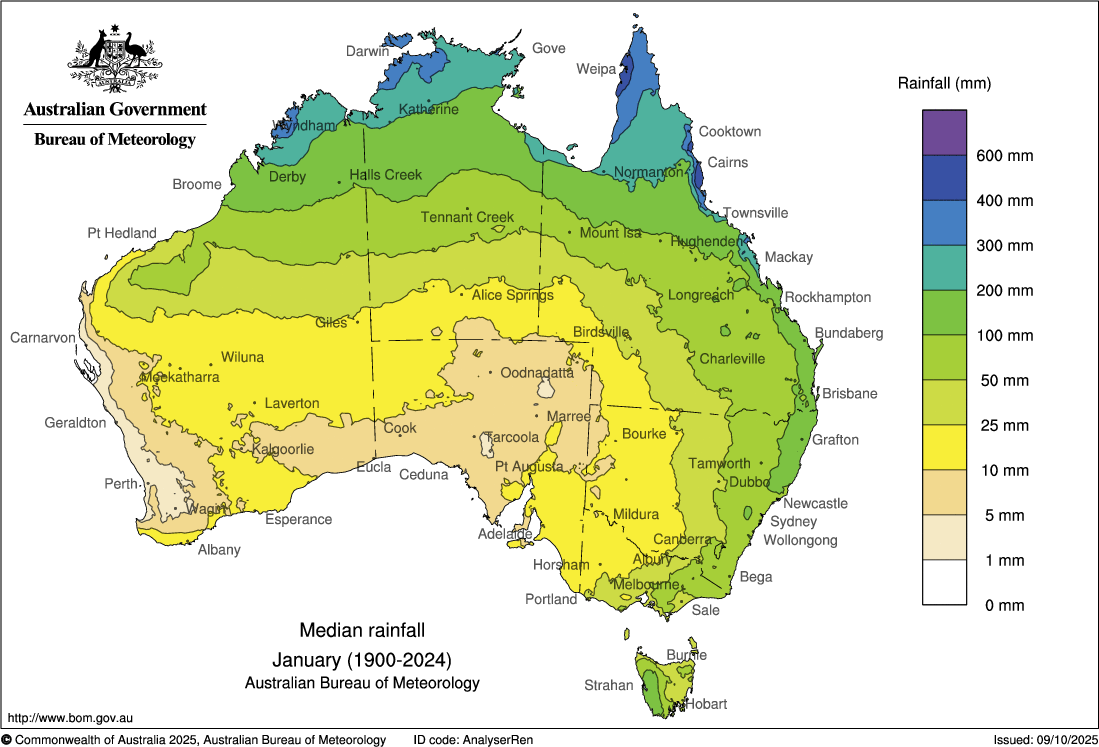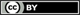# Rainfall percentiles maps

These rainfall percentile maps show the monthly, seasonal, multi-seasonal and annual rainfall percentile distributions across Australia.Product code: IDCJCM0016

## What do the maps show?

These maps show the monthly, seasonal, multi-seasonal and annual rainfall percentiles for Australia. These percentiles have been calculated over the 120-year period 1900 to 2019. The rainfall percentile gives the ranking, in percentage terms, of all recorded rainfall from lowest to highest.

For a given rainfall percentile, the corresponding rainfall amount is threshold below which that percentage of all recorded years falls. For example, consider a particular location on the 10th percentile map for January. The rainfall amount (or rainfall value) at that location marks the threshold for the lowest 10% of records. In other words, 10% of the January rainfall totals recorded at this location over the period 1900 to 2019 have been less than the value shown on the map. Similarly, a particular location on the 90th percentile map marks the threshold of the lowest 90% of records, equivalent to the highest 10%. This means that, at this location, 10% of the records are greater than the rainfall value shown on the map.

Percentiles give the likely occurrence of particular events. If you have a rainfall value in the 30th percentile, this means that there is roughly a 30% chance of any rainfall value for that site being equal to or below this value. Similarly, if you have a rainfall value in the 80th percentile, there is roughly an 80% chance that any rainfall value for this site will be equal to or below this value.

Highest and lowest percentile maps give us an idea of the extremes experienced in various regions and tell us how variable the rainfall can be. For example, parts of northern Australia experience very high rainfall totals when tropical cyclones affect the coast. This leads to a high value of the 100% percentile.

These maps show the average distribution of rainfall percentiles at different times across Australia. Looking at these maps, you will notice that the distribution of rainfall percentiles is similar to the distribution of average rainfall totals.

If you compare increasing rainfall percentile maps, you will notice that the rainfall values get higher, while still retaining a similar rainfall distribution pattern.

## How are the values calculated?

Percentiles are used as an indicator of the likelihood of a particular event. Percentiles are related to deciles and are expressed as a number out of 100 (similar to a percentage). The percentile refers to the ranking of a particular value relative to all of the values for that site. For example, if there were 100 rainfall values recorded for a site, we could place them in order from the least to the most rainfall. Of these values, the 10th lowest value would be called the 10th percentile and the 20th lowest value the 20th percentile. This is applied right up until the 100th percentile, which includes all of the values that have been measured for that site, and is equal to the maximum rainfall value.Unless otherwise noted, all maps, graphs and diagrams in this page are licensed under the Creative Commons Attribution 4.0 International Licence